# Job sequencing (algorithm, time complexity and example) in Operating System

In this article, we will study about the concept of job sequencing with its algorithm, time complexity and example.
Submitted by Shivangi Jain, on June 22, 2018

## Job sequencing

Job sequencing is the set of jobs, associated with the job i where deadline di >= 0 and profit pi > 0. For any job i the profit is earned if and only if the job is completed by its deadline. To complete a job, one has to process the job on a machine for one unit of time. Only one machine is available for processing the jobs.

Steps for performing job sequencing with deadline using greedy approach is as follows:

1. Sort all the jobs based on the profit in an increasing order.
2. Let α be the maximum deadline that will define the size of array.
3. Create a solution array S with d slots.
4. Initialize the content of array S with zero.
5. Check for all jobs.
1. If scheduling is possible a lot ith slot of array s to job i.
2. Otherwise look for location (i-1), (i-2)...1.
3. Schedule the job if possible else reject.
6. Return array S as the answer.
7. End.

### Algorithm for job sequencing

Input: A is the array of jobs with deadline and profit S array will be the output.

```    1. Begin
2. Sort all the jobs based on profit Pi so
3. P1 > P2 > P3 …………………………….>=Pn
4. d = maximum deadline of job in A
5. Create array S[1,…………………,d]
6. For i=1 to n do
7. Find the largest job x
8. For j=i to 1
9. If ((S[j] = 0) and (x deadline<= d))
10. Then
11. S[x] = i;
12. Break;
13. End if
14. End for
15. End for
16. End
```

### Time complexity

Job sequencing problems has the time complexity of O(n2).

Example:

Given a set of 9 jobs where each job has a deadline and profit associated to it .Each job takes 1 unit of time to complete and only one job can be scheduled at a time. We earn the profit if and only if the job is completed by its deadline. The task is to find the maximum profit and the number of jobs done.

```    Jobs	 Profit	  Deadline
J1	        85	        5
J2	        25	        4
J3	        16	        3
J4	        40	        3
J5	        55	        4
J6	        19	        5
J7	        92	        2
J8	        80	        3
J9	        15	        7
```

Step 1: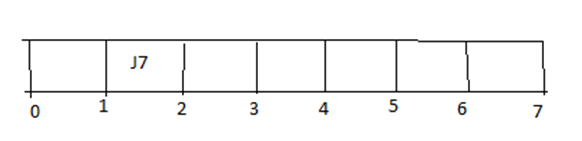Step 2: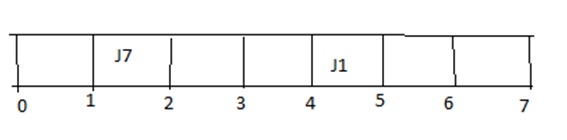Step 3: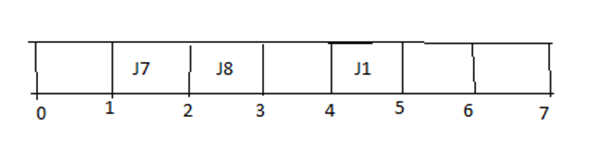Step 4: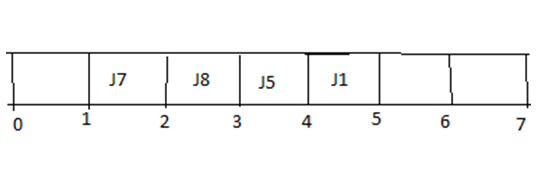Step 5: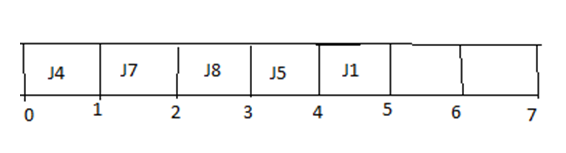Step 6: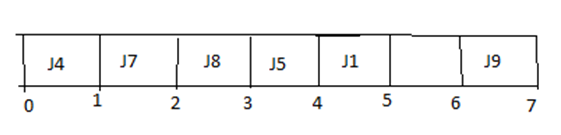So, the maximum profit = 40 + 92 + 80 + 55 + 85 + 15 = 367

Preparation

What's New

Top Interview Coding Problems/Challenges!